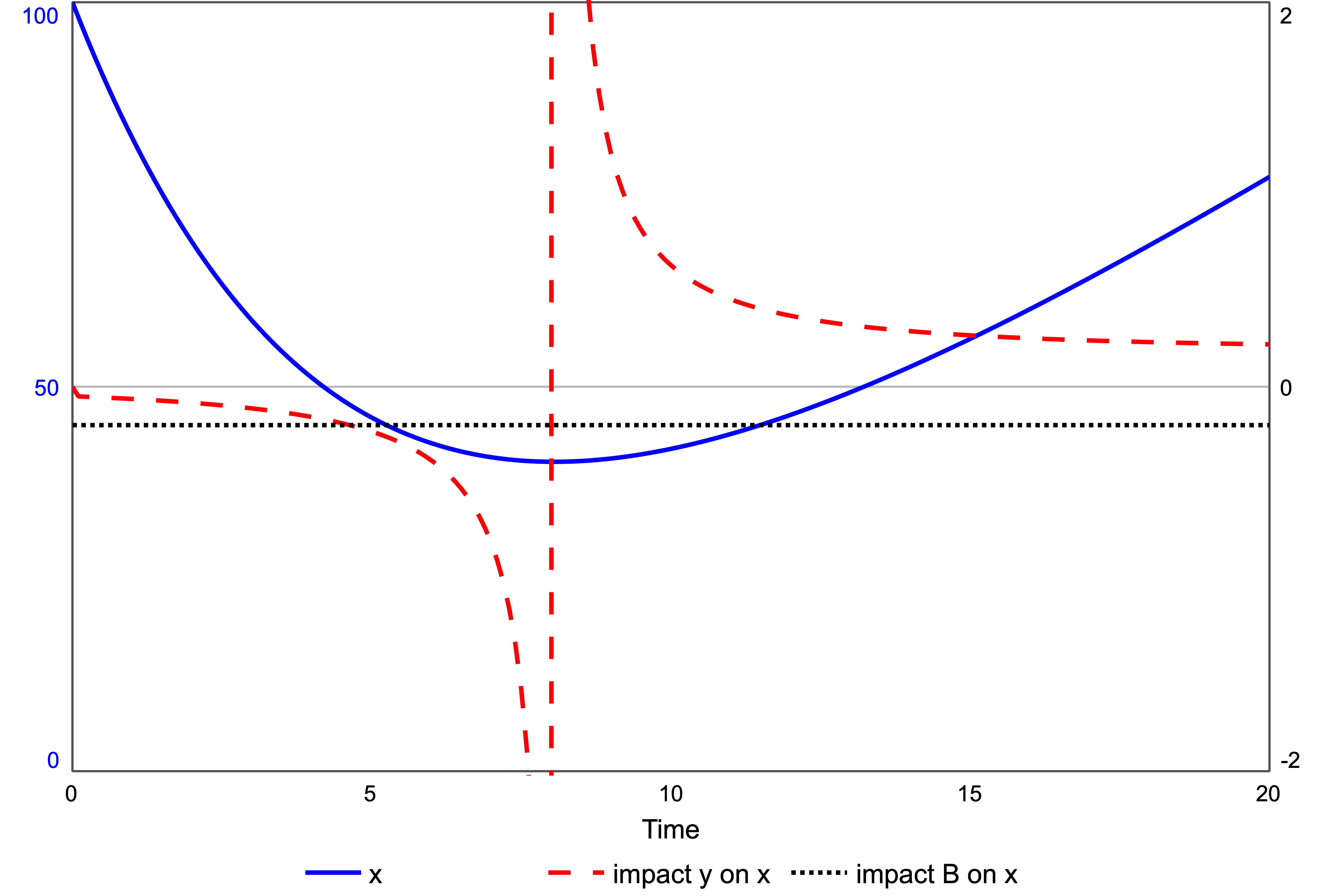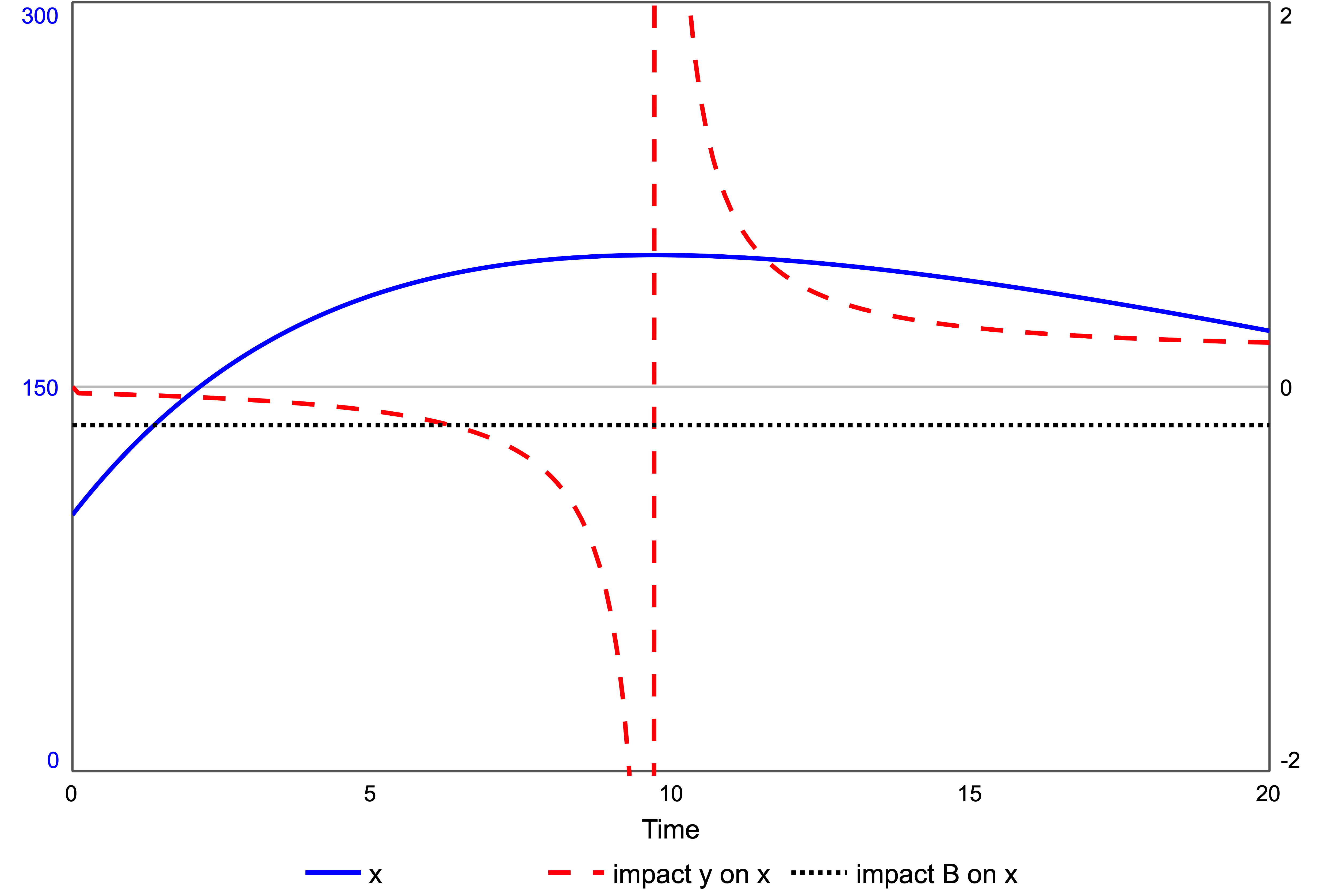Force and Impact

In mechanics, force produces acceleration in an object. The same concept can be used in system dynamics to describe how any time-varying signal can cause acceleration in a stock – a dynamic variable. That signal may be exogenous or come from another stock. In the Newtonian Interpretive Framework, the effect of the force is measured by impact, the ratio of the acceleration of the stock to its rate of change – net flow; the equivalent of velocity. If x is a stock, the impact of all forces on the stock gives:

Thus, force and impact have different sign conventions depending on the net flow dx/dt.

To compare these differences, imagine you are sitting at the origin where there is a positive force on nearby objects – repelling force. If the object is at rest, the force accelerates it, causing it to move away. If the object is already moving away, the force still accelerates it, moving it away faster. The positive force produces positive acceleration. From the impact equation (1), the impact of the force on the stock is positive as both numerator and denominator are positive.

But if an object is moving toward you, the positive force tries to slow the object in order to stop it and send it away again. Thus, the object decelerates. A positive force now has a negative effect on the object. From equation (1) the impact of the force on the object is negative as the denominator dx/dt is negative for an object move toward the origin.

Therefore, a positive force may have a positive impact, or a negative impact, depending on whether the object is moving away, or moving towards, the origin. Impact picks up the effect of the force on the object.

The same comparison between force and impact applies in system dynamics. Consider a stock y exerting a positive force on stock x, figure1. A positive force has g > 0. Stock x is also subject to a negative force, the balancing feedback loop B, a resistive force.

The flow g is set to a constant, g = 1, so that y increases uniformly, starting from zero. Thus, the force of y on x is constant and positive, equal to one. With x starting at 100, the force from the balancing loop is much larger than that of y; thus, x declines, figure 2. Thus, dx/dt < 0 and the impact of y on x is negative, the dashed line in figure 2. A positive force, but a negative impact. The impact of loop B is also negative as the loop is balancing, dotted line figure 2.Figure 2. Stock x, left axis. The impacts of y and loop B on x. Positive force, g = 1.

Initially, loop B exerts the strongest force on x, indicated by its impact having greater magnitude that that from y. At time 4.5, the impact of y exceeds the balancing loop. It is this exogenous force that is responsible for bringing the stock to rest, a momentary equilibrium, at time 8.0 .

After this minimum point, the stock x starts to increase again, driven by the force from y now acting as an accelerator. A positive force with a positive impact, indicated in figure 2 by the dashed line jumping from negative to positive. Impact from y on x is momentarily infinite at x‘s minimum, as dx/dt = 0.

From time = 8 onward, the balancing loop B is resisting the positive force from y, but it remains smaller. As time tends to infinity, the impact of y on x tends to the absolute value of the impact of B, with x tending to uniform increase.

The situation is reversed if the force is negative – an attracting force. In the model of figure 1, a negative force occurs if g < 0 . Let g = -1 with y starting at 50. Stock x increases to a maximum, then declines, figure 3.Figure 3. Stock x, left axis. The impacts of y and loop B on x. Positive force, g = -1.

Figure 3 shows the impact of y on x is the same as that for the positive force, figure 2. Up to the maximum point, the negative force has a negative impact, slowing the growth of x. After the maximum, the negative force has a positive impact, accelerating the decline of x.

An advantage of measuring the force by impact is that the sign of the impact matches the type of behaviour, plus mean acceleration, minus meaning deceleration. It follows that impact is related to the curvature in the graph of the stock. A further advantage of impact is that if the force comes from a first-order loop, impact is positive, with a negative sign for balancing loops. Thus, impact is a natural way of measuring force in system dynamics.

Notes

 Although a first-order balancing loop can bring a stock to rest (equilibrium), it only does so in the limit as time tends to infinity. It requires an exogenous effect, or a higher-order loop, to bring a stock to rest in a finite time.

 The force from y to x can also be made negative by altering the connection between y and the flow into x such that a rising y gives falling flow, e.g. f1 = 1/y.

Reinforcing Loops and Growth

I have often seen the following causal loop used in system dynamics presentations to explain a reinforcing loop:

Figure 1: Causal Loop Diagram of Simple Birth Process

The argument then runs: the more population, the more births, hence more in the population; thus the population grows. Fortunately, I never hear people reverse the argument: the fewer in the population, the fewer births, hence fewer in the population! Clearly not true. If the only process on the population is births, it must grow. In fact, the population will grow even if births stay constant.

The problem with a causal loop diagram is that it does not give any information about which variables are stocks, and thus accumulate. Every causal loop must have at least one stock; otherwise, time does not advance, and there is a circular definition. In the above, Population is a stock, and births are a flow. Thus, the argument should read: the more population, the more births, thus the more are added to the population.

The + sign on the link to births means “same way” that is the births change the same way as the population. If the population goes up, then so do births; if the population goes down so do the births.

However, the + sign on Population means “add to”. If the births go up, more are added to the population; however, if births go down, less are added to the population. But the population still increases because it is being added to.

Figure 2: Stock-Flow Diagram of Simple Birth Process

Now it is clear that the stock Population is being added to. The reinforcing loop describes a process where the births being added to the population is increasing. Thus the population growth accelerates:

Figure 3: Accelerating Growth for 1-Stock Reinforcing Loop (per capita rate of growth 0.2 per unit time)

The reinforcing loop gives accelerating growth. Acceleration is the characteristic behaviour of a reinforcing loop containing only one stock. Acceleration is portrayed as a curve becoming more vertical, figure 3. If, however, the reinforcing loop is broken:

Figure 4: Stock-Flow diagram of Constant Growth

then the resulting growth has no acceleration and appears as a straight line:

Figure 5: Uniform Growth of a Stock with a Constant Flow (births = 1 person per year)

The absence of the loop does not mean the absence of growth, but the absence of acceleration. If there is growth, then it is uniform.

In this way a reinforcing loop (figure 2) can be pictured as a force on a stock, producing acceleration (figure 3) – the equivalent of Newton’s second law of motion. The absence of a loop (figure 4) is like the absence of a force – Newton’s first law of motion – producing uniform growth (figure 5). This analogy is an example of the Newtonian Interpretative Framework which lies at the heart of Sociomechanics, which seeks to explain social behaviour using ideas from mechanics.